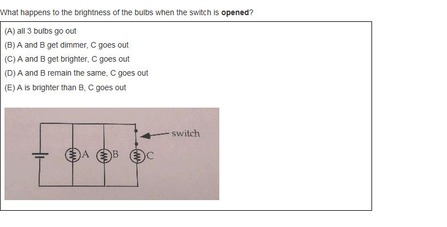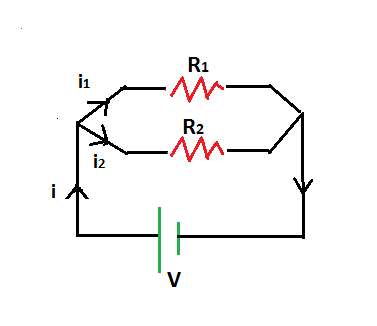# What happens to the brightness of the bulbs when the switch is opened? (A) all 3 bulbs go out (B)...

## Question:

What happens to the brightness of the bulbs when the switch is opened?

(A) all 3 bulbs go out

(B) A and B get dimmer, C goes out

(C) A and B get brighter, C goes out

(D) A and B remain the same, C goes out

(E) A is brighter than B, C goes out## Parallel Combination of Resistors:

When two resistances are connected in a parallel, the current flowing through them gets divided while the voltages across them remain constant. In the diagram below we see that the current i is divided into two parts which pass through the two resistances.

The voltage across the two resistances is the same and is equal to the voltage of the battery V.Given:

In the given diagram when the switch is opened, no current flows through the resistance C thus it goes out.

Now, we see that the resistances...

Become a Study.com member to unlock this answer! Create your account